# Permeance: Definition, Units & Coefficient

Contents

## What is Permeance?

Permeance is defined as a measure of the ease with which magnetic flux can be admitted through a material or magnetic circuit. Permeance is the reciprocal of reluctance. Permeance is directly proportional to the magnetic flux and is denoted by the letter P.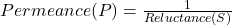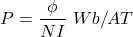From the above equation we can say that the quantity of magnetic flux for a number of ampere-turns is depended on permeance.

In terms of magnetic permeability, permeance is given by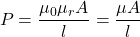Where,

•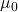= Permeability of free space (vacuum) =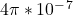Henry/meter
•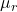= Relative permeability of a magnetic material
•= Length of the magnetic path in meter
•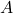= Cross sectional area in square meters (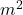)

In an electric circuit, conductance is the degree to which an object conducts electricity; similarly, permeance is the degree to which the magnetic flux conducts in a magnetic circuit. Therefore, permeance is larger for larger cross-sections and smaller for smaller cross-sections. This concept of permeance in a magnetic circuit is analogous to conductance in an electrical circuit.

## Reluctance vs Permeance

The differences between reluctance and permeance have been discussed in the table below.

## Permeance Units

The units of permeance are Weber per ampere-turns (Wb/AT) or Henry.

## Total Magnetic Flux (ø) and Permeance (P) in a Magnetic Circuit

The magnetic flux is given by

(1)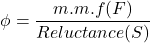but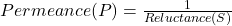By using this relation into equation (1) we get,

(2)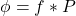Now, the total magnetic flux i.e.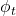for an entire magnetic circuit is the sum of air gap flux i.e.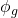and leakage flux i.e.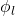.

(3)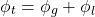As we know that the permeance for a magnetic circuit is given by

(4)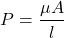From the equation (4), we can say that for greater the cross-section area and permeability, and the shorter the magnetic path length, the greater the permeance (i.e. the smaller the reluctance or magnetic resistance).

Now permeance i.e. Pt for the entire magnetic circuit is the sum of air gap permeance i.e. Pg and leakage permeance i.e. Pf which is caused by leakage magnetic flux ().

(5)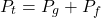When there is more than one air gap space in the magnetic path, the total permeance is expressed as a sum of air gap permeance and the leakage permeance of each magnetic path space i.e.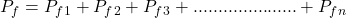.

Therefore, the total permeance is

(6)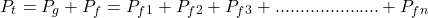## Relation Between Permeance and Leakage Coefficient

The leakage coefficient is the ratio of total magnetic flux generated by the magnet in the magnetic circuit to the air gap flux. It is denoted by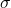.

(7)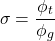From equation (2) i.e.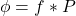, put this into equation (7) we get,

(8)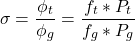Now in equation (8) the ratio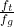is the magneto motive force loss coefficient which is close to 1 and Pt = Pg + Pf , Put these into equation (8) we get,

(9)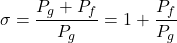Now for more than one air gap space in a magnetic path, the leakage coefficient is given by,

(10)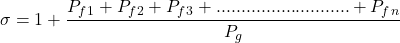The above equation indicates the relationship between permeance and leakage coefficient.

## Permeance Coefficient

Permeance coefficient is defined as the ratio of magnetic flux density to magnetic field strength at the operating slope of the B-H curve.

It is used to express the “operating point” or “operating slope” of the magnet on the load line or B-H curve. Thus the permeance coefficient is very useful in designing magnetic circuits. It is denoted by PC.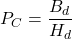Where,

•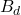= Magnetic flux density at the operating point of B-H curve
•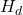= Magnetic field strength at the operating point of B-H curve

In the above graph, the straight line OP passing between the origin and theandpoints on the B-H curve (also called as demagnetization curve) is called the permeance line and the slope of the permeance line is the permeance coefficient PC.

For the only single magnet, that is when there is no other permanent magnet (hard magnetic material) or soft magnetic material placed nearby, we can calculate the permeance coefficient PC from the shape and the dimensions of the magnet. Therefore, we can say that the permeance coefficient is a figure of merit for a magnet.

### What Is Unit Permeance?

Permeance coefficient PC is given by

(11)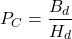But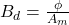and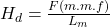put these into equation (11) we get,

(12)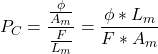But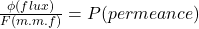, put this into equation (12) we get,

(13)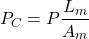Now, when the length of the magnet i.e.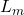and cross-section area i.e.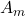is equal to the size of the unit, then in this condition

(14)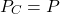Thus, the permeance coefficient PC is equivalent to Permeance P. It can be called as unit permeance.

Want To Learn Faster? 🎓
Get electrical articles delivered to your inbox every week.
No credit card required—it’s 100% free.